## Tuesday, March 5, 2019

### Plotting method for Mk model with intraspecific polymorphism

I just pushed a new phytools update to GitHub. It primarily involves the addition of an S3 plotting method to the function `fitpolyMk` that I described a couple of days ago, but also fixes a small bug in `fitpolyMk` itself.

Just to review, this function fits an extended Mk to data that include intraspecific polymorphism. The basic idea is that if we imagine two states, A and B, evolution from A to B must occur through the intermediate polymorphic condition of A + B. If we extend this logic to more than two states then we must decide if evolution is ordered (i.e., AA + BBB + CC, and so on) or unordered, in which case we might suppose that multistate polymorphism (e.g., A + B + C) can also occur with some probability.

When I first tried to envision a plotting method for this function I tried to design a circular graph similar to what I previously developed for `fitMk`. For instance, here is a beta attempt I posted to my Facebook page: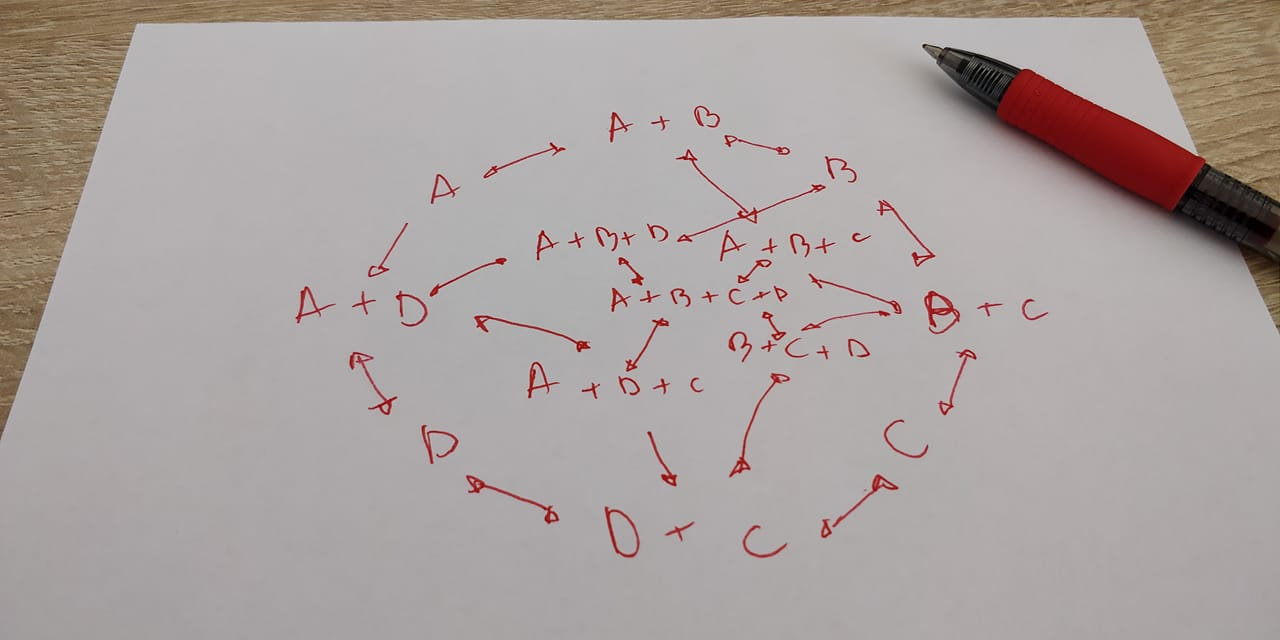Unfortunately, this type of design becomes very messy & not so easy to interpret for even a relatively small number of character states.

Instead, I ultimately settled on a circular design for the ordered model, but a more-or-less 'vertical' design for the unordered polymorphic transition model, which I'll show in a moment.

Here, once again I'm going to demonstrate the fitting & plotting methods using simulated polymorphic discrete character data. Perhaps it's also worth noting that we don't have to observe all possible polymorphic conditions in our data to fit a model in which these conditions can exist!

First, let's load our updated version of phytools:

``````library(phytools)
packageVersion("phytools")
``````
``````##  '0.6.68'
``````

Now we'll work with a set of simulated datasets. For now, there is no way of specifying order in the 'ordered' model: it is assumed to be alphanumeric. (I intend to update this later, but it seems like a relatively minor inconvenience.)

Let's start by looking at our first data vector & tree, `x` & `tree`, respectively, and then plotting these data on the phylogeny:

``````tree
``````
``````##
## Phylogenetic tree with 200 tips and 199 internal nodes.
##
## Tip labels:
##  t103, t104, t197, t198, t74, t75, ...
##
## Rooted; includes branch lengths.
``````
``````x
``````
``````##  t103  t104  t197  t198   t74   t75   t53   t85  t132  t185  t186   t64
##     A     A     A     A     B     B     B   A+B     A     A     A     B
##   t87   t94  t136  t137  t148  t149   t27   t78   t79  t187  t188  t163
##     B     B     B     B     B     B     B     B A+B+C   A+B     B     B
##  t164   t38   t39   t72   t73    t4  t116  t117   t43   t42  t139  t140
##     B     B     B     B     B     B     B   A+B     B     B     B     B
##  t195  t196  t165   t41  t109  t179  t180  t146  t147  t100   t99  t174
##     B     B     B     B   B+C     B     B   B+C   B+C   B+C   A+C     B
##  t175   t50   t60   t61   t44   t15   t16    t7  t152  t153  t199  t200
##     B   B+C     C     B     B     B   A+C     A     B     B     B     B
##  t159  t160   t59   t90   t91   t34  t110  t111   t82   t22   t70   t71
##     B     B     B     B     B     B     C     C     C   B+C   A+B     B
##   t21   t30   t31   t20   t62   t63  t127  t128   t95   t96   t46  t129
##     B     A     B   B+C     B     B     A     A     B     B     B   B+C
##  t130  t114  t115   t67   t23   t24   t57   t58   t11  t154  t176  t177
## A+B+C   A+C     C   A+C     B     B     B     B   A+B     B     B     B
##  t138  t191  t192  t178    t6   t29  t125  t126   t45   t54  t189  t190
##     B     B     B     B     C     B     B   B+C   A+B   A+B     B     B
##  t112  t113   t65   t66   t32   t33  t181  t182  t161  t162  t121  t105
##     B     B     B     B     B     B     B     B     B     B     B     B
##  t106  t157  t158   t92   t93   t76   t77   t84  t150  t151  t143    t3
##     B   B+C   B+C     B A+B+C     B     B     B     B     B     B     C
##   t12   t13   t51   t52  t107  t108   t37   t25   t26    t2    t9   t10
##     B     B     C     B   B+C     B   A+B   A+B     A     B   B+C     B
##   t18   t19    t8   t28   t36   t48   t86  t155  t156   t81   t88   t89
##     A   A+B     C     B     B A+B+C     C     C     C     A     A     A
##  t172  t173   t49   t17  t141  t142  t131  t101  t102   t35   t68   t69
##     A     A     A     C   A+B   A+B   A+B     A     A     A     A     A
##  t166  t167  t122  t123  t119  t120   t14   t55   t56   t47  t124  t183
##     A   A+B   A+C     C     C     C     C     A A+B+C     C     C     C
##  t184  t168  t169  t134  t135   t83  t170  t171   t40  t144  t145   t80
##     C     C     C     C     C     C     A     A   A+C     C     A     C
##  t193  t194  t133  t118    t5    t1   t97   t98
##     A     A     A     A     C     B     C     C
## Levels: A A+B A+B+C A+C B B+C C
``````
``````plotTree(tree,ftype="off",lwd=1,type="fan")
X<-strsplit(setNames(as.character(x),names(x)),"+",fixed=TRUE)
pies<-matrix(0,Ntip(tree),3,dimnames=list(tree\$tip.label,c("A","B","C")))
for(i in 1:Ntip(tree))
pies[tree\$tip.label[i],X[[tree\$tip.label[i]]]]<-rep(1/length(X[[tree\$tip.label[i]]]),
length(X[[tree\$tip.label[i]]]))
tiplabels(pie=pies,piecol=c("black","yellow","red"),cex=0.35)
legend(x="topleft",legend=c("A","B","C"),pt.cex=2,pch=21,
pt.bg=c("black","yellow","red"))
``````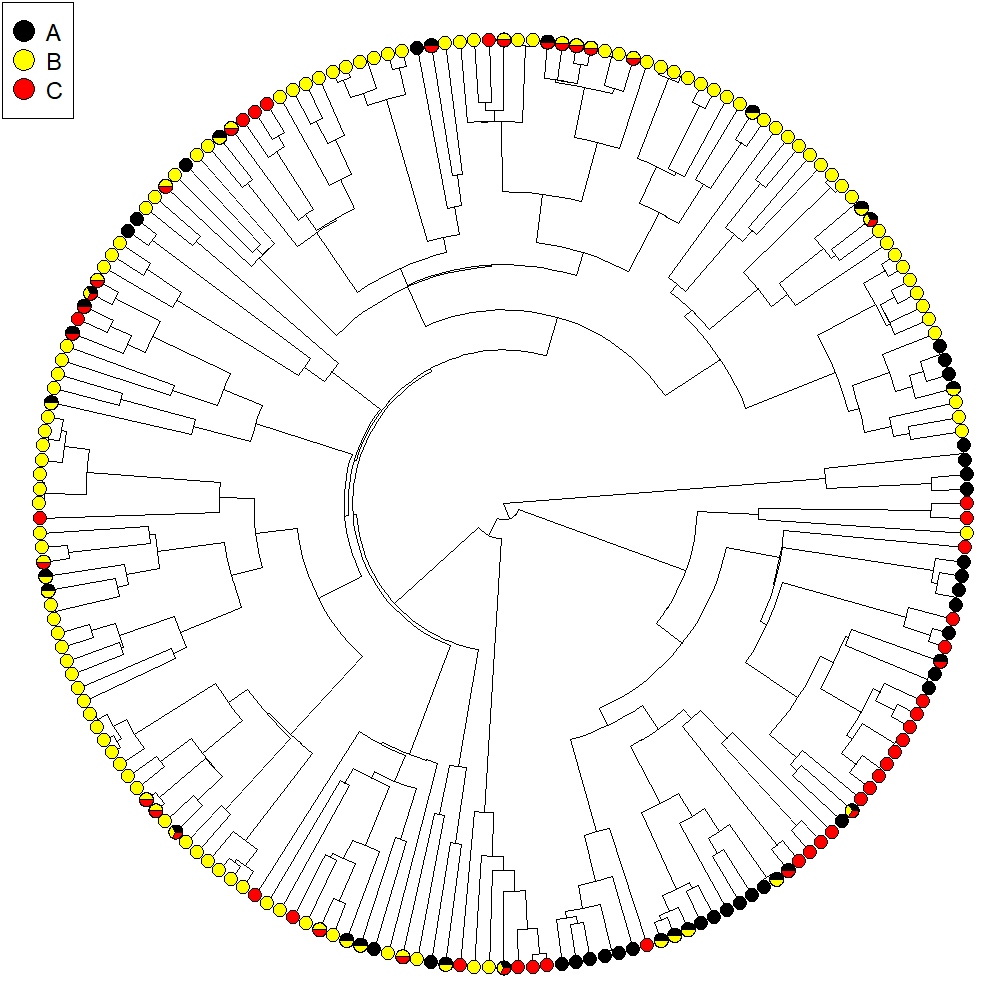Next, what I'm going to do is fit a few different models to the data and compare them, just as I did in my previous post. Note that for character `x` we must fit an 'unordered' model because the multistate polymorphic condition (`A+B+C` in this case) exists in our data.

``````ard.unordered<-fitpolyMk(tree,x,model="ARD")
``````
``````##
## This is the design matrix of the fitted model. Does it make sense?
##
##       A B  C A+B A+C B+C A+B+C
## A     0 0  0   1   3   0     0
## B     0 0  0   5   0   7     0
## C     0 0  0   0   9  11     0
## A+B   2 6  0   0   0   0    13
## A+C   4 0 10   0   0   0    15
## B+C   0 8 12   0   0   0    17
## A+B+C 0 0  0  14  16  18     0
``````
``````ard.unordered
``````
``````## Object of class "fitpolyMk".
##
## Evolution was modeled as 'unordered' using the "ARD" model.
##
## Fitted (or set) value of Q:
##               A         B         C       A+B        A+C        B+C
## A     -0.958476  0.000000  0.000000  0.958476   0.000000   0.000000
## B      0.000000 -1.871278  0.000000  0.654131   0.000000   1.217148
## C      0.000000  0.000000 -7.424279  0.000000   7.424279   0.000000
## A+B    3.519568  2.210898  0.000000 -5.730466   0.000000   0.000000
## A+C    6.287675  0.000000 32.728264  0.000000 -42.921115   0.000000
## B+C    0.000000  6.194701  2.114481  0.000000   0.000000 -13.379396
## A+B+C  0.000000  0.000000  0.000000  0.000000   8.116189  10.849789
##            A+B+C
## A       0.000000
## B       0.000000
## C       0.000000
## A+B     0.000000
## A+C     3.905176
## B+C     5.070214
## A+B+C -18.965978
##
## Fitted (or set) value of pi:
##         A         B         C       A+B       A+C       B+C     A+B+C
## 0.1428571 0.1428571 0.1428571 0.1428571 0.1428571 0.1428571 0.1428571
##
## Log-likelihood: -198.978338
##
## Optimization method used was "nlminb"
``````
``````sym.unordered<-fitpolyMk(tree,x,model="SYM")
``````
``````##
## This is the design matrix of the fitted model. Does it make sense?
##
##       A B C A+B A+C B+C A+B+C
## A     0 0 0   1   2   0     0
## B     0 0 0   3   0   4     0
## C     0 0 0   0   5   6     0
## A+B   1 3 0   0   0   0     7
## A+C   2 0 5   0   0   0     8
## B+C   0 4 6   0   0   0     9
## A+B+C 0 0 0   7   8   9     0
``````
``````sym.unordered
``````
``````## Object of class "fitpolyMk".
##
## Evolution was modeled as 'unordered' using the "SYM" model.
##
## Fitted (or set) value of Q:
##               A         B         C       A+B       A+C       B+C
## A     -3.775788  0.000000  0.000000  2.254469  1.521320  0.000000
## B      0.000000 -1.592209  0.000000  0.646719  0.000000  0.945491
## C      0.000000  0.000000 -6.519037  0.000000  4.204605  2.314431
## A+B    2.254469  0.646719  0.000000 -2.901187  0.000000  0.000000
## A+C    1.521320  0.000000  4.204605  0.000000 -6.429092  0.000000
## B+C    0.000000  0.945491  2.314431  0.000000  0.000000 -5.719308
## A+B+C  0.000000  0.000000  0.000000  0.000000  0.703167  2.459386
##           A+B+C
## A      0.000000
## B      0.000000
## C      0.000000
## A+B    0.000000
## A+C    0.703167
## B+C    2.459386
## A+B+C -3.162553
##
## Fitted (or set) value of pi:
##         A         B         C       A+B       A+C       B+C     A+B+C
## 0.1428571 0.1428571 0.1428571 0.1428571 0.1428571 0.1428571 0.1428571
##
## Log-likelihood: -224.236358
##
## Optimization method used was "nlminb"
``````
``````transient.unordered<-fitpolyMk(tree,x,model="transient")
``````
``````##
## This is the design matrix of the fitted model. Does it make sense?
##
##       A B C A+B A+C B+C A+B+C
## A     0 0 0   2   2   0     0
## B     0 0 0   2   0   2     0
## C     0 0 0   0   2   2     0
## A+B   1 1 0   0   0   0     2
## A+C   1 0 1   0   0   0     2
## B+C   0 1 1   0   0   0     2
## A+B+C 0 0 0   1   1   1     0
``````
``````transient.unordered
``````
``````## Object of class "fitpolyMk".
##
## Evolution was modeled as 'unordered' using the "transient" model.
##
## Fitted (or set) value of Q:
##               A         B         C       A+B       A+C       B+C
## A     -1.918232  0.000000  0.000000  0.959116  0.959116  0.000000
## B      0.000000 -1.918232  0.000000  0.959116  0.000000  0.959116
## C      0.000000  0.000000 -1.918232  0.000000  0.959116  0.959116
## A+B    3.876625  3.876625  0.000000 -8.712366  0.000000  0.000000
## A+C    3.876625  0.000000  3.876625  0.000000 -8.712366  0.000000
## B+C    0.000000  3.876625  3.876625  0.000000  0.000000 -8.712366
## A+B+C  0.000000  0.000000  0.000000  3.876625  3.876625  3.876625
##            A+B+C
## A       0.000000
## B       0.000000
## C       0.000000
## A+B     0.959116
## A+C     0.959116
## B+C     0.959116
## A+B+C -11.629875
##
## Fitted (or set) value of pi:
##         A         B         C       A+B       A+C       B+C     A+B+C
## 0.1428571 0.1428571 0.1428571 0.1428571 0.1428571 0.1428571 0.1428571
##
## Log-likelihood: -208.806632
##
## Optimization method used was "nlminb"
``````
``````er.unordered<-fitpolyMk(tree,x,model="ER")
``````
``````##
## This is the design matrix of the fitted model. Does it make sense?
##
##       A B C A+B A+C B+C A+B+C
## A     0 0 0   1   1   0     0
## B     0 0 0   1   0   1     0
## C     0 0 0   0   1   1     0
## A+B   1 1 0   0   0   0     1
## A+C   1 0 1   0   0   0     1
## B+C   0 1 1   0   0   0     1
## A+B+C 0 0 0   1   1   1     0
``````
``````er.unordered
``````
``````## Object of class "fitpolyMk".
##
## Evolution was modeled as 'unordered' using the "ER" model.
##
## Fitted (or set) value of Q:
##               A         B         C       A+B       A+C       B+C
## A     -2.467694  0.000000  0.000000  1.233847  1.233847  0.000000
## B      0.000000 -2.467694  0.000000  1.233847  0.000000  1.233847
## C      0.000000  0.000000 -2.467694  0.000000  1.233847  1.233847
## A+B    1.233847  1.233847  0.000000 -3.701541  0.000000  0.000000
## A+C    1.233847  0.000000  1.233847  0.000000 -3.701541  0.000000
## B+C    0.000000  1.233847  1.233847  0.000000  0.000000 -3.701541
## A+B+C  0.000000  0.000000  0.000000  1.233847  1.233847  1.233847
##           A+B+C
## A      0.000000
## B      0.000000
## C      0.000000
## A+B    1.233847
## A+C    1.233847
## B+C    1.233847
## A+B+C -3.701541
##
## Fitted (or set) value of pi:
##         A         B         C       A+B       A+C       B+C     A+B+C
## 0.1428571 0.1428571 0.1428571 0.1428571 0.1428571 0.1428571 0.1428571
##
## Log-likelihood: -233.190591
##
## Optimization method used was "nlminb"
``````

As I did earlier, we can similarly compare among models using AIC:

``````data.frame(transition_model=c("ARD","SYM","transient","ER"),
logLik=c(logLik(ard.unordered),logLik(sym.unordered),
logLik(transient.unordered),logLik(er.unordered)),
k=c(attr(AIC(ard.unordered),"df"),attr(AIC(sym.unordered),"df"),
attr(AIC(transient.unordered),"df"),attr(AIC(er.unordered),"df")),
AIC=c(AIC(ard.unordered),AIC(sym.unordered),AIC(transient.unordered),
AIC(er.unordered)))
``````
``````##   transition_model    logLik  k      AIC
## 1              ARD -198.9783 18 433.9567
## 2              SYM -224.2364  9 466.4727
## 3        transient -208.8066  2 421.6133
## 4               ER -233.1906  1 468.3812
``````

Now we can also visualize these fitted models. Here are plots of the two best-fitting models: `"ARD"` and the `"transient"` model.

``````plot(ard.unordered,main="Fitted ARD \'unordered\' model:")
``````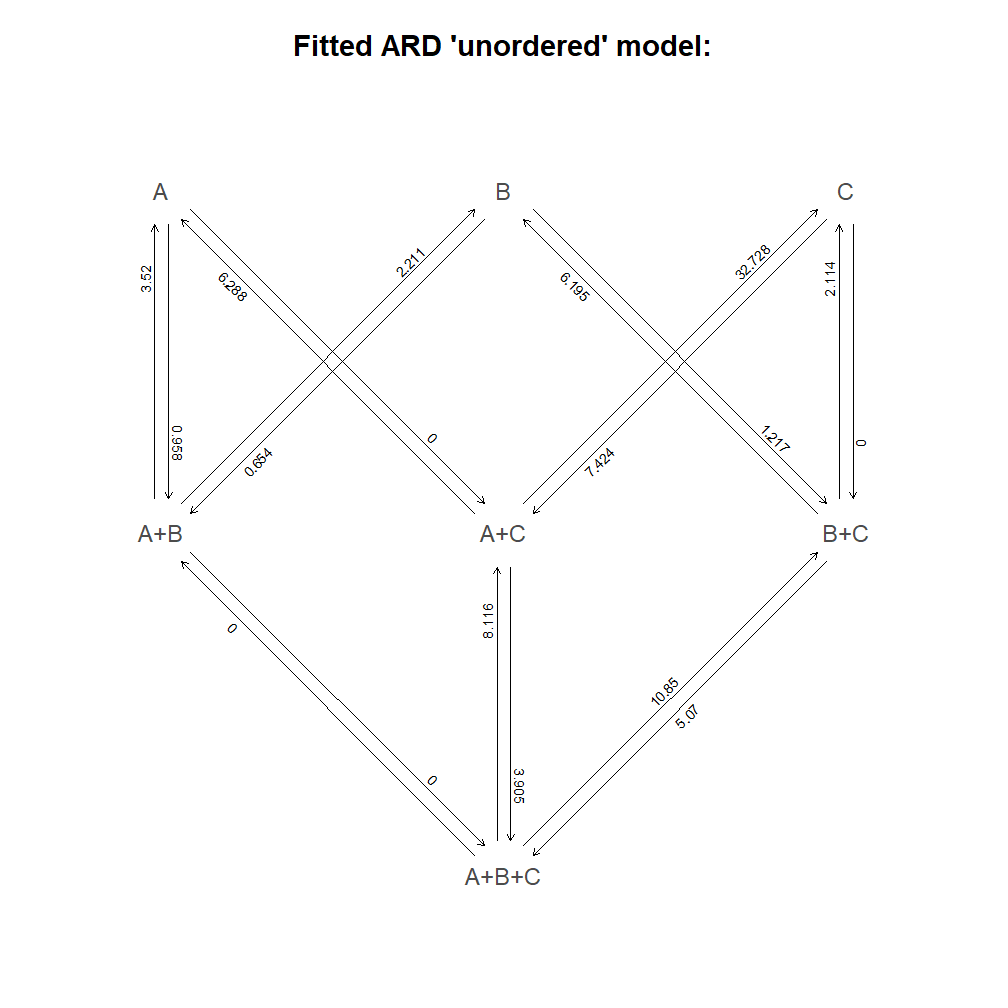``````plot(transient.unordered,main="Fitted \'transient\' model:")
``````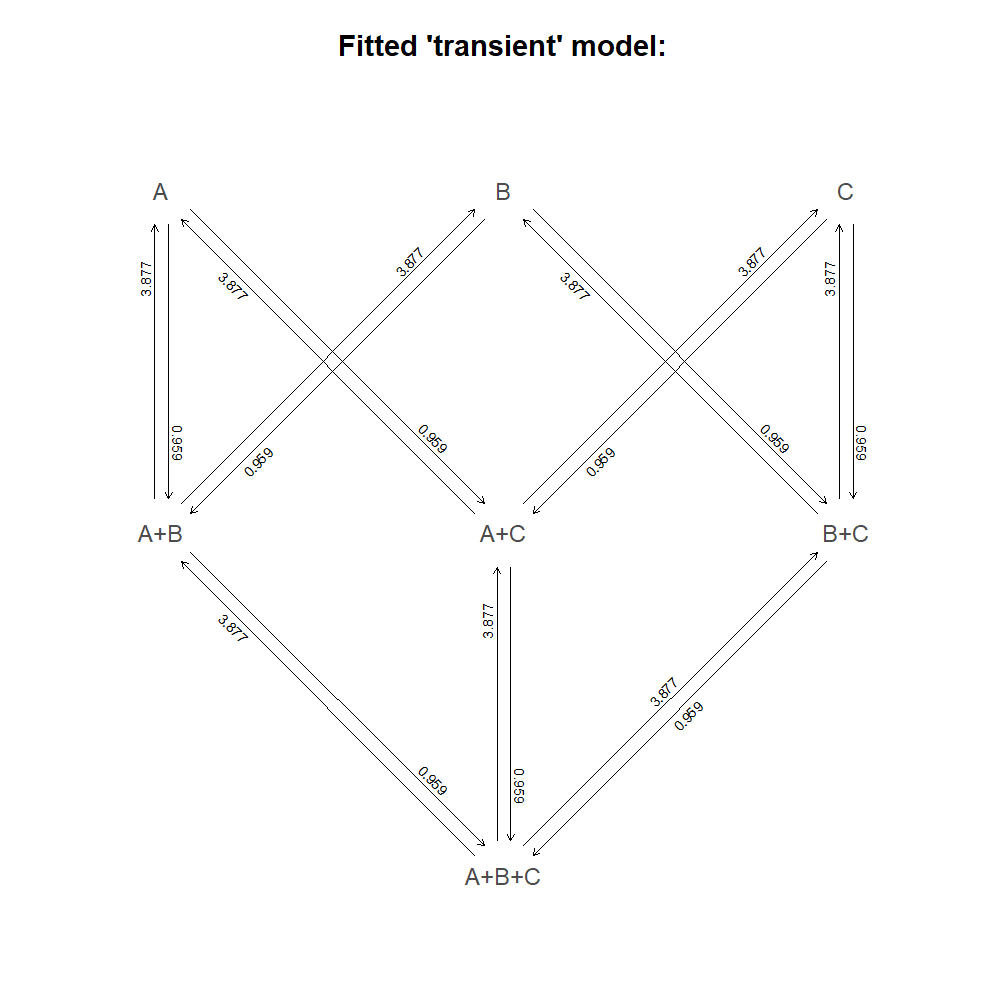Pretty neat, right?

Next, let's look at a 4-state character with intraspecific polymorphism. Here (for simplicity) I'll just presume an `"ER"` process and ask whether the data support the 'ordered' or 'unordered' model:

``````## plot data
plotTree(tree,ftype="off",lwd=1,type="fan")
X<-strsplit(setNames(as.character(y),names(y)),"+",fixed=TRUE)
pies<-matrix(0,Ntip(tree),4,dimnames=list(tree\$tip.label,c("A","B","C","D")))
for(i in 1:Ntip(tree))
pies[tree\$tip.label[i],X[[tree\$tip.label[i]]]]<-rep(1/length(X[[tree\$tip.label[i]]]),
length(X[[tree\$tip.label[i]]]))
tiplabels(pie=pies,piecol=c("black","yellow","red","blue"),cex=0.35)
legend(x="topleft",legend=c("A","B","C","D"),pt.cex=2,pch=21,
pt.bg=c("black","yellow","red","blue"))
``````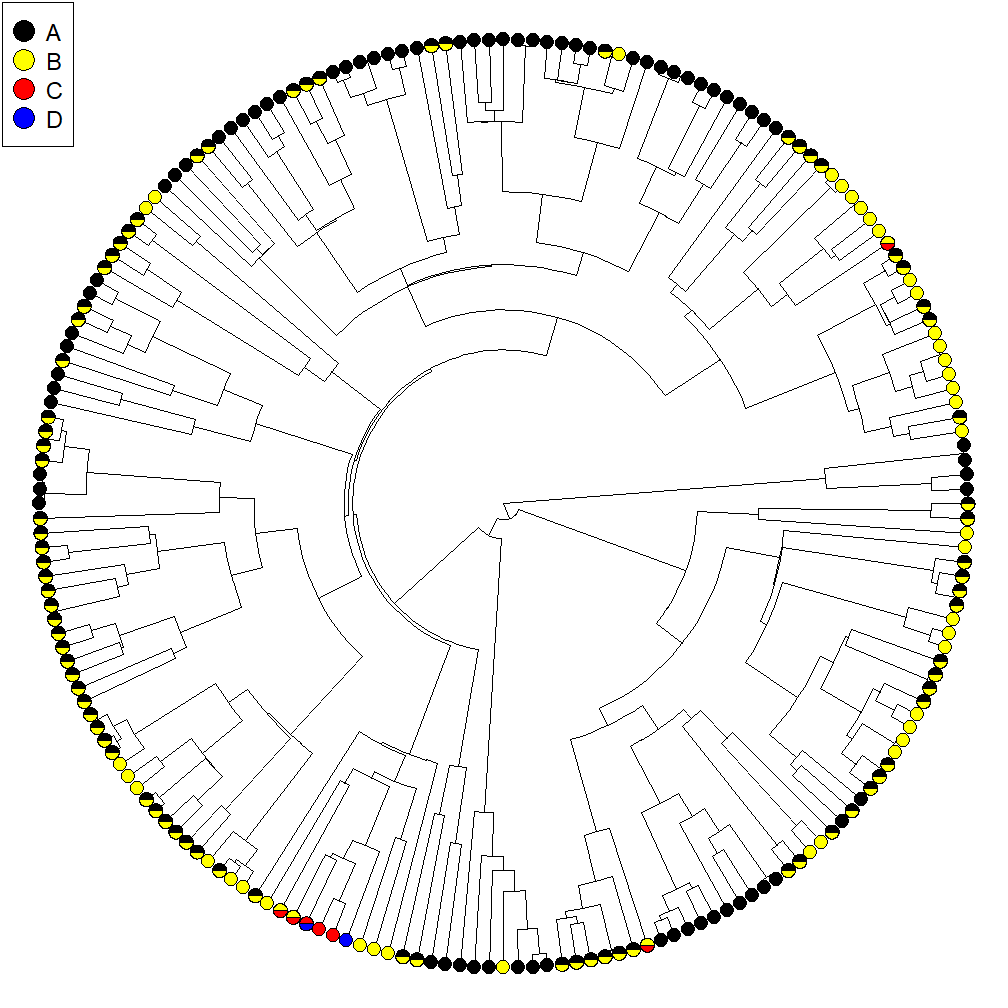``````## fit models:
er.ordered<-fitpolyMk(tree,y,model="ER",ordered=TRUE)
``````
``````##
## This is the design matrix of the fitted model. Does it make sense?
##
##     A A+B B B+C C C+D D
## A   0   1 0   0 0   0 0
## A+B 1   0 1   0 0   0 0
## B   0   1 0   1 0   0 0
## B+C 0   0 1   0 1   0 0
## C   0   0 0   1 0   1 0
## C+D 0   0 0   0 1   0 1
## D   0   0 0   0 0   1 0
``````
``````er.unordered<-fitpolyMk(tree,y,model="ER")
``````
``````##
## This is the design matrix of the fitted model. Does it make sense?
##
##         A B C D A+B A+C A+D B+C B+D C+D A+B+C A+B+D A+C+D B+C+D A+B+C+D
## A       0 0 0 0   1   1   1   0   0   0     0     0     0     0       0
## B       0 0 0 0   1   0   0   1   1   0     0     0     0     0       0
## C       0 0 0 0   0   1   0   1   0   1     0     0     0     0       0
## D       0 0 0 0   0   0   1   0   1   1     0     0     0     0       0
## A+B     1 1 0 0   0   0   0   0   0   0     1     1     0     0       0
## A+C     1 0 1 0   0   0   0   0   0   0     1     0     1     0       0
## A+D     1 0 0 1   0   0   0   0   0   0     0     1     1     0       0
## B+C     0 1 1 0   0   0   0   0   0   0     1     0     0     1       0
## B+D     0 1 0 1   0   0   0   0   0   0     0     1     0     1       0
## C+D     0 0 1 1   0   0   0   0   0   0     0     0     1     1       0
## A+B+C   0 0 0 0   1   1   0   1   0   0     0     0     0     0       1
## A+B+D   0 0 0 0   1   0   1   0   1   0     0     0     0     0       1
## A+C+D   0 0 0 0   0   1   1   0   0   1     0     0     0     0       1
## B+C+D   0 0 0 0   0   0   0   1   1   1     0     0     0     0       1
## A+B+C+D 0 0 0 0   0   0   0   0   0   0     1     1     1     1       0
``````
``````data.frame(transition_model=c("ER","ER"),
ordered=c("yes","no"),
logLik=c(logLik(er.ordered),logLik(er.unordered)),
k=c(attr(AIC(er.ordered),"df"),attr(AIC(er.unordered),"df")),
AIC=c(AIC(er.ordered),AIC(er.unordered)))
``````
``````##   transition_model ordered    logLik k      AIC
## 1               ER     yes -149.8048 1 301.6096
## 2               ER      no -189.3070 1 380.6141
``````

Clearly here the 'ordered' model is much better supported than the 'unordered' model here, but for fun let's still plot each of them. Here, I'll change the line colors using `par` (I plan to add this as a built-in feature of the plotting method in future versions):

``````par(fg=make.transparent("blue",0.5))
plot(er.ordered,main="Fitted ER \'ordered\' model:",lwd=2)
``````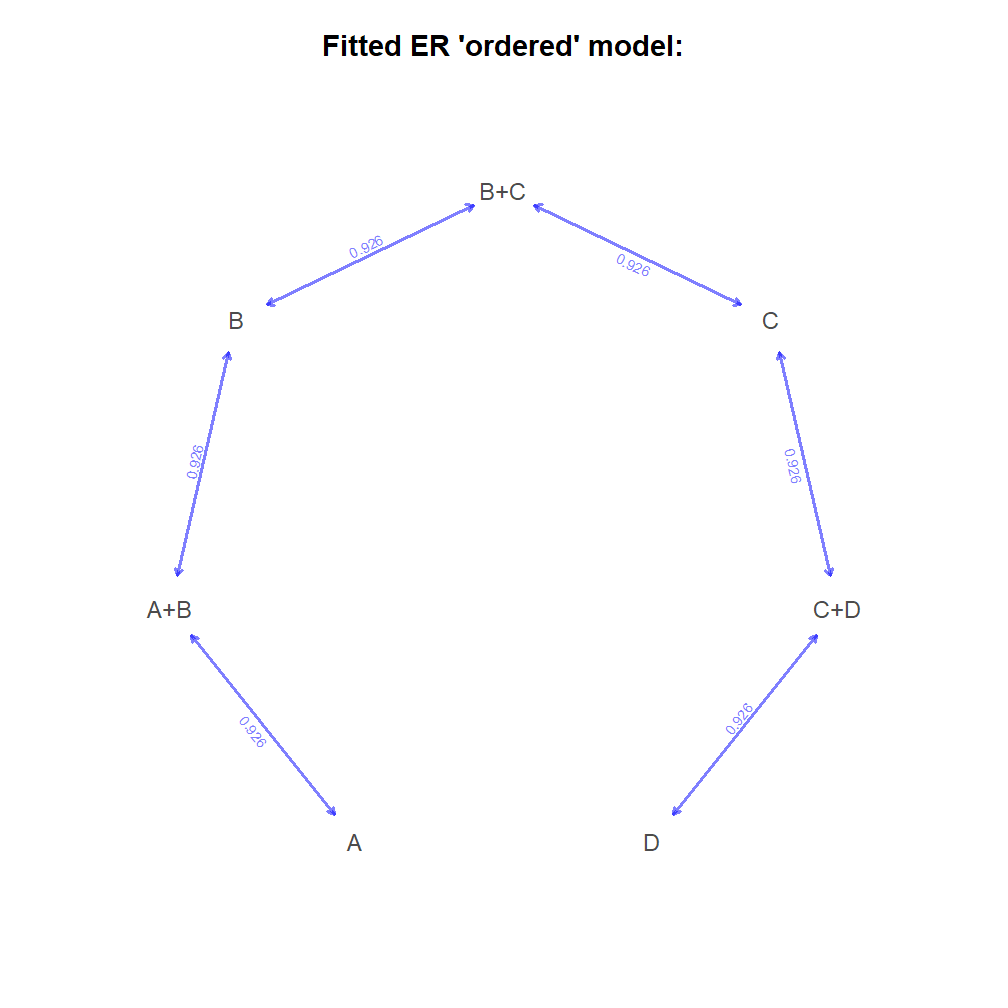``````par(fg=make.transparent("blue",0.5))
plot(er.unordered,main="Fitted ER \'unordered\' model:",lwd=2)
``````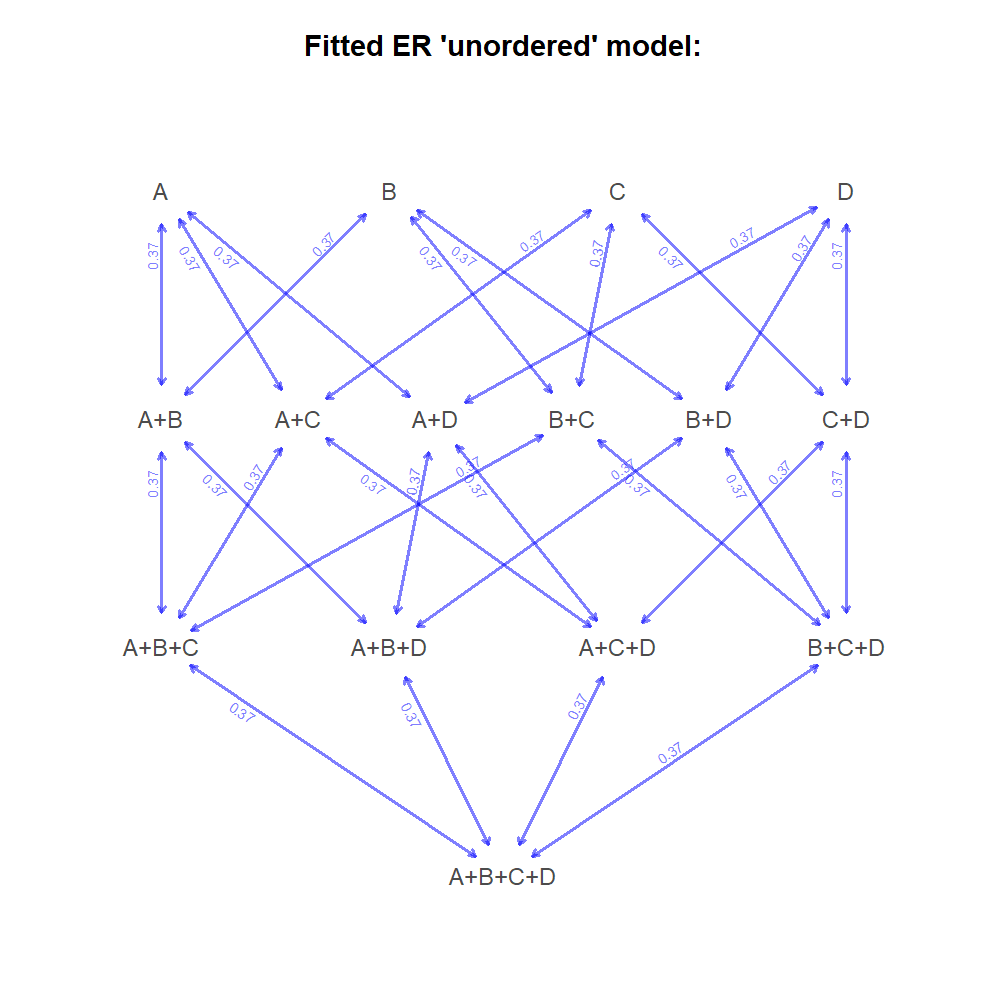``````par(fg="black")
``````

Finally, let's take a look at a 5-state polymorphic trait. Once again, for simplicity, I'll presume a `"transient"` model & compare 'ordered' and 'unordered' versions of it. I'll also test these against the simpler `"ER"` equivalents:

``````z
``````
``````## t103 t104 t197 t198  t74  t75  t53  t85 t132 t185 t186  t64  t87  t94 t136
##    D    D    D    D  B+C  B+C    B    C    C    C    C    C    C    C    C
## t137 t148 t149  t27  t78  t79 t187 t188 t163 t164  t38  t39  t72  t73   t4
##    C    C    C    A    B    B    B    B    B    B  A+B    B    B    B    D
## t116 t117  t43  t42 t139 t140 t195 t196 t165  t41 t109 t179 t180 t146 t147
##    C    C  C+D    D    D    D    D    D    D    B    B    B    B    B    B
## t100  t99 t174 t175  t50  t60  t61  t44  t15  t16   t7 t152 t153 t199 t200
##    B    A    C    C    B    B    B  C+D    C    C    C  B+C    C  B+C  B+C
## t159 t160  t59  t90  t91  t34 t110 t111  t82  t22  t70  t71  t21  t30  t31
##  B+C  B+C    B    B    B    B  B+C    B    B  B+C    B    B    D    C  B+C
##  t20  t62  t63 t127 t128  t95  t96  t46 t129 t130 t114 t115  t67  t23  t24
##    D    D    D    D    D    C    C    C    C    C    D    C    C    C  C+D
##  t57  t58  t11 t154 t176 t177 t138 t191 t192 t178   t6  t29 t125 t126  t45
##  B+C    B  B+C    D    D    D  C+D    D    D    D    D    D    D    D    D
##  t54 t189 t190 t112 t113  t65  t66  t32  t33 t181 t182 t161 t162 t121 t105
##  D+E    E    E  B+C    C    C    C    D    C    C    C    C    C    C    C
## t106 t157 t158  t92  t93  t76  t77  t84 t150 t151 t143   t3  t12  t13  t51
##  B+C    C    C    C    C  A+B    B  C+D  C+D    D  C+D    B  B+C    B    D
##  t52 t107 t108  t37  t25  t26   t2   t9  t10  t18  t19   t8  t28  t36  t48
##    C  C+D    C    B    B  A+B    B  A+B  A+B    B    A    C    C  C+D    C
##  t86 t155 t156  t81  t88  t89 t172 t173  t49  t17 t141 t142 t131 t101 t102
##    C    C    C    D    D  D+E    D    D    D    D    D    D    D    D    D
##  t35  t68  t69 t166 t167 t122 t123 t119 t120  t14  t55  t56  t47 t124 t183
##    D    D    D    D    D    D    D    D    D    D    D    D    D  D+E    D
## t184 t168 t169 t134 t135  t83 t170 t171  t40 t144 t145  t80 t193 t194 t133
##    D    D    D    D    D    D  C+D  C+D    E    D    D    D    D    D    D
## t118   t5   t1  t97  t98
##    D    D    C    C    C
## Levels: A A+B B B+C C C+D D D+E E
``````
``````transient.unordered<-fitpolyMk(tree,z,model="transient",quiet=TRUE)
transient.ordered<-fitpolyMk(tree,z,model="transient",ordered=TRUE,quiet=TRUE)
er.unordered<-fitpolyMk(tree,z,model="ER",quiet=TRUE)
er.ordered<-fitpolyMk(tree,z,model="ER",ordered=TRUE,quiet=TRUE)
data.frame(transition_model=c("transient","transient","ER","ER"),
ordered=c("no","yes","no","yes"),
logLik=c(logLik(transient.unordered),logLik(transient.ordered),
logLik(er.unordered),logLik(er.ordered)),
k=c(attr(AIC(transient.unordered),"df"),attr(AIC(transient.ordered),"df"),
attr(AIC(er.unordered),"df"),attr(AIC(er.ordered),"df")),
AIC=c(AIC(transient.unordered),AIC(transient.ordered),
AIC(er.unordered),AIC(er.ordered)))
``````
``````##   transition_model ordered    logLik k      AIC
## 1        transient      no -230.5158 2 465.0316
## 2        transient     yes -202.2833 2 408.5667
## 3               ER      no -297.6168 1 597.2337
## 4               ER     yes -227.6106 1 457.2212
``````

Tbe transient-ordered seems to be best, but let's plot it & the unordered model too:

``````par(fg=make.transparent("blue",0.5))
plot(transient.unordered,cex.traits=0.8,main="Unordered \'transient\' model:")
``````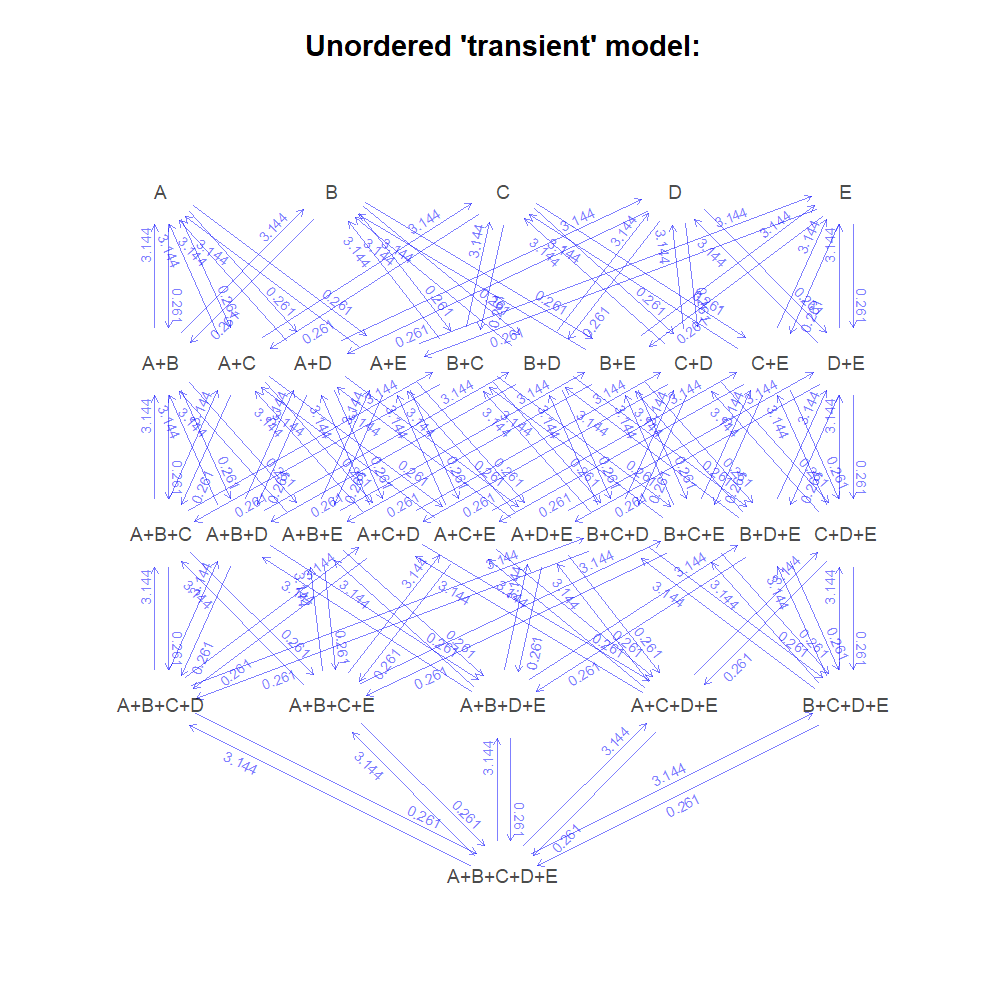``````plot(transient.ordered,cex.traits=0.8,main="Ordered \'transient\' model:")
``````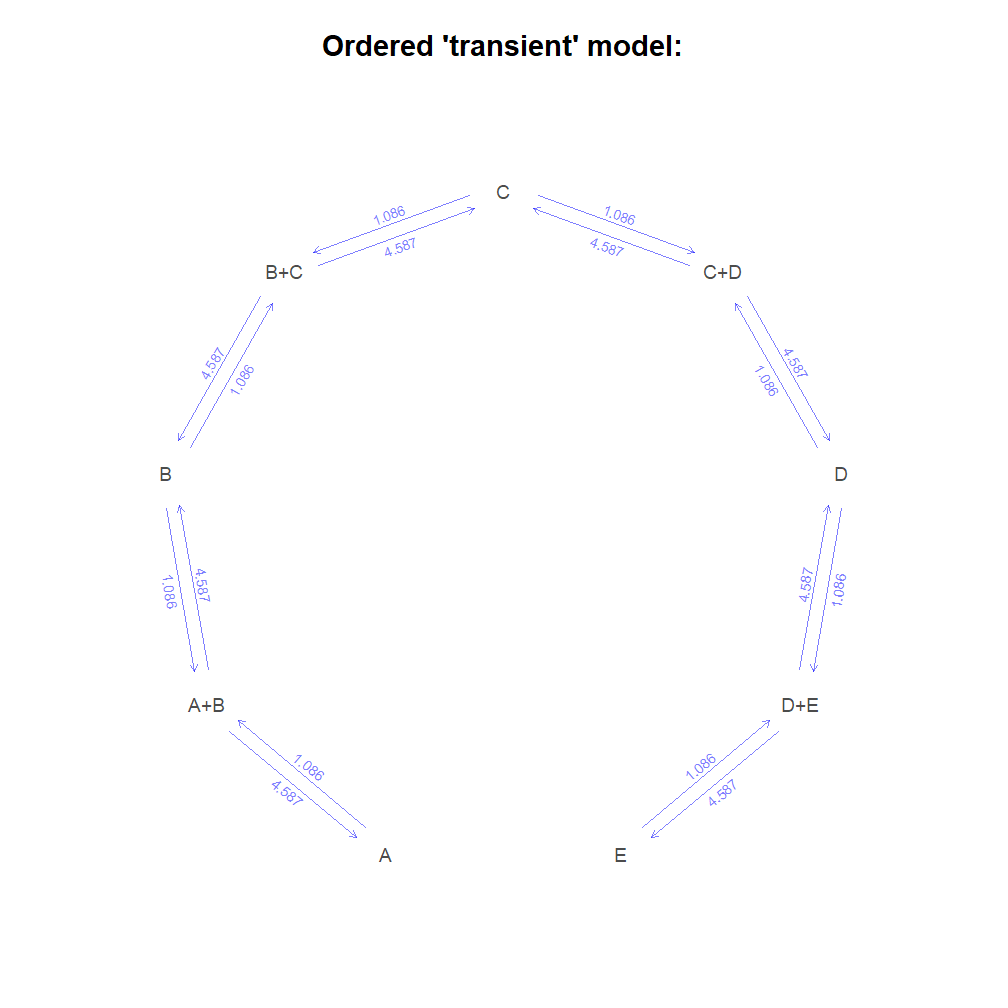Cool. Also, the best-fit models (of the ones we considered) happen to have been the generating models in each case. Here's how the phylogeny & data were simulated:

``````## pure-birth phylogeny
tree<-pbtree(n=200,scale=1)

## 4-state 'transient' unordered model
Q<-matrix(0,7,7)
rownames(Q)<-colnames(Q)<-c("A","B","C","A+B","A+C","B+C","A+B+C")
Q[1,]<-c(0,0,0,1,1,0,0)
Q[2,]<-c(0,0,0,1,0,1,0)
Q[3,]<-c(0,0,0,0,1,1,0)
Q[4,]<-c(4,4,0,0,0,0,1)
Q[5,]<-c(4,0,4,0,0,0,1)
Q[6,]<-c(0,4,4,0,0,0,1)
Q[7,]<-c(0,0,0,4,4,4,0)
diag(Q)<--rowSums(Q)
x<-sim.Mk(tree,Q)

## 4-state 'ER' ordered
Q<-matrix(c(-1,1,0,0,0,0,0,
1,-2,1,0,0,0,0,
0,1,-2,1,0,0,0,
0,0,1,-2,1,0,0,
0,0,0,1,-2,1,0,
0,0,0,0,1,-2,1,
0,0,0,0,0,1,-1),7,7,byrow=TRUE)
rownames(Q)<-colnames(Q)<-c("A","A+B","B","B+C","C","C+D","D")
y<-sim.Mk(tree,Q)

## 5-state 'ER' ordered
Q<-matrix(c(-1,1,0,0,0,0,0,0,0,
4,-8,4,0,0,0,0,0,0,
0,1,-2,1,0,0,0,0,0,
0,0,4,-8,4,0,0,0,0,
0,0,0,1,-2,1,0,0,0,
0,0,0,0,4,-8,4,0,0,
0,0,0,0,0,1,-2,1,0,
0,0,0,0,0,0,4,-8,4,
0,0,0,0,0,0,0,1,-1),9,9,byrow=TRUE)
rownames(Q)<-colnames(Q)<-c("A","A+B","B","B+C","C","C+D","D","D+E","E")
z<-sim.Mk(tree,Q)
``````

That's all for now!

1.Liam, all this reminds me of Polymorphism Parsimony, which was explored in the 1960s-1970s and was mentioned in my phylogenies book. Any relation?

1.Hi Joe. The implicit model is similar, but it looks like in polymorphism parsimony the derived character state can only be acquired once, whereas here there is no such implicit restriction (unless we specify it). - Liam

2.This is very impressive. Do you think there will be a way to expand this method to also model the relative proportion of different states? So not just A+B, but 40% A, 60% B? I guess such proportions would make the function very computationally challenging, right?

1.Mark - this would be really cool, right? Unfortunately, within the current framework its a bit complicated....

3.Hi, Liam!

It's Patricia, I don't know if you remember me but we met at the Maldonado course (I am the girl who works with climbing plants). I was trying to plot the pie charted phylogeny for a 4-state character and kept getting an error right after trying to plot the little pie charts. Can you maybe help me? Here's the code with the error message:

###
> plotTree(climber.tree,ftype="off",lwd=1,type="fan")
> X<-strsplit(setNames(as.character(Mechs),names(Mechs)),"+",fixed=TRUE)
> pies<-matrix(0,Ntip(climber.tree),4,dimnames=list(climber.tree\$tip.label,c("1","2","3","4")))
> for(i in 1:Ntip(climber.tree))
+ pies[climber.tree\$tip.label[i],X[[climber.tree\$tip.label[i]]]]<-rep(1/length(
+ X[[climber.tree\$tip.label[i]]]),length(X[[climber.tree\$tip.label[i]]]))
> tiplabels(pie=pies,piecol=c("black","yellow","red","blue"),cex=0.2)

Error in seq.default(x[i], x[i + 1], length = n) :
'to' must be a finite number

> legend(x="topleft",legend=c("Leaning","Root climbing","Main stem twining",
+ "Prehensile/Twining structures"),pt.cex=2,pch=21,
+ pt.bg=c("black","yellow","red","blue"))
###

Everything else but the pie charts is plotted. Any light you can give me will be much appreciated.

Thank you so much!

Best,

1.Hi Patricia.

Yes, I remember you.

Does your data contain polymorphism (i.e., more than one state at some tips containing a "+" to separate the two or more states)? That is what the strsplit line is for.

Does the pies matrix look right? I.e., does it contain a 1.0 under the corresponding state for every tip - and a 0.5 0.5 (if two states) for every bimorphic taxon?

Other than these simple checks I would need to see the tree & data. Please feel free to email me the files & a minimum script to reproduce the error & I would be happy to look into it.

- Liam

2.Hi Liam,

Well, it seems like I'm gonna have to email you the files, the pies matrix has only zeros in it and I have no idea what happened.

I'll send it to you in a moment.

Thank you!

Note: due to the very large amount of spam, all comments are now automatically submitted for moderation.